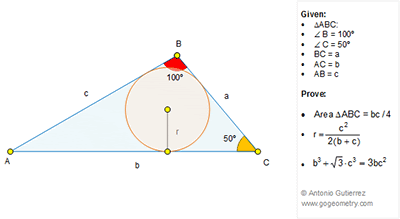## Thursday, November 24, 2016

### Geometry Problem 1288 Triangle, 30-50-100 Degrees, Area, Inradius, Metric Relations, Measurement

Geometry Problem. Post your solution in the comment box below.
Level: Mathematics Education, High School, Honors Geometry, College.

This entry contributed by Sumith Peiris, Moratuwa, Sri Lanka.

Click on the figure below to view more details of problem 1288.1.Problem 1288
Draw BK perpendicular in AC,then BK=AB/2=c/2 and AK=√3c/2.
Let area triangle ABC is (ABC)=(AC.BK)/2=bc/4.
Let s=(a+b+c)/2 then (ABC)=sr.So r=(ABC)/s=bc/4s, suffices to show that bc/4s=c^2/2(b+c) or b/(a+b+c)=c/(b+c) or b^2=c(a+c).
In extension of the AB passes point D such that BD=BC=a,then triangle ABC is similar with triangle ACD so AB/AC=AC/AD or c/b=b/(a+c) or b^2=c(a+c).So r=(c^2)/(2(b+c)).
APOSTOLIS MANOLOUDIS 4 HIGH SCHOOL KORYDALLOS PIRAEUS GREECE

2.https://goo.gl/photos/mJcfykipaSNAr2en6

Draw CD such that angle BCD= 50 . D is on AB extended (see sketch).
1. S(ABC) =1/2.AC.AB.sin(30)= b.c/4
2. Note that angle BDC= 50 and tri CBD is isosceles => BD=BC= a
Triangle ABC and ACD are similar => AC/AD=AB/AC= c/b => AD= b^2/c
BD= AD-AB= b^2/c-c= a
AB/AC=BC/CD=c/b => CD=a.b/c= b(b^2/c^2-1)
Inradius r= S(ABC)/2p where 2p= a+b+c= b+b^2/c
. r=b.c/4p= bc/2/(b+b^2/c) => r=c^2/(2(b+c))
3. Apply cosine formula to triangle ACD
Replace CD=b(b^2/c^2-1) ; AD= b^2/c in (1) and simplifying we have
. (b^2/c^2-1)^2=1+b^2/c^2-(b/c).sqrt(3)
This equation will simplify to b^3+sqrt(3).c^3= 3.b.c^2

3.Draw a perpendicular from B to AC and meet it at D
Consider the 30-60-90 triangle ABD
Since AB = c, BD = c/2, AD = Sqrt(3)c/2
Hence Area of ABC = bc/4 ---------- (1)

Draw an Angle Bisector for m(AB,BC) and let it meet AC at E
From Angle bisector theorem, CE = ab/a+c and AE = bc/a+c
Since triangle BEC is isosceles (50,50,80), BE = CE = ab/a+c ---------(2)
If you observe, triangle ABC is similar to AEB
Hence BE/c=a/b => BE = ac/b --------------(3)
From (2) and (3) ab/a+c = ac/b
hence a = b^2-c^2/c -------(4)
As we know semi-perimeter for triangle ABC, S = a+b+c/2
substituting value of 'a' from (4), we get S = b^2+bc/2c
We know, Area ABC in terms of S and r = S.r = bc/4 (from (1))
=> (b^2+bc/2c ).r = bc/4
=> r= c^2/2(b+c) ----------- (5)

Now consider the right triangle BED
We know BD = c/2 , BE = b^2-c^2/2b (Substitute for 'a' in ac/b)
and ED = AD - AE = Sqrt(3)bc-2c^2/2b
Applying pythogorus, we get BE^2 = BD^2+ED^2
=> (b^2-c^2/b)^2 = (Sqrt(3)bc-2c^2/2b)^2 + c^2/4
Simplifying, we get b^3+Sqrt(3)c^3=3b^2c^2 ----------------(6)